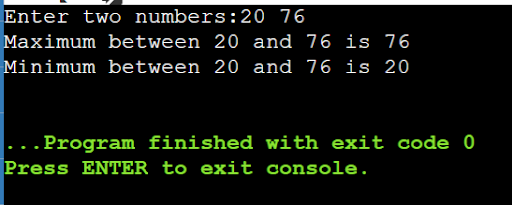Write a program in C++, to find maximum and minimum of two numbers using conditional operator.

Write a program in C++, to find maximum and minimum of two numbers using conditional operator.

verified

#include<iostream>

using namespace std;

int main()

{

int num1, num2, max, min;

cout<<"Enter two numbers:";

cin>>num1>>num2;

max = (num1 > num2) ? num1 : num2;

min = (num1 < num2) ? num1 : num2;

cout<<"Maximum between "<<num1<<" and "<<num2<<" is "<<max<<endl;

cout<<"Minimum between "<<num1<<" and "<<num2<<" is "<<min<<endl;

return 0;

}

Output::